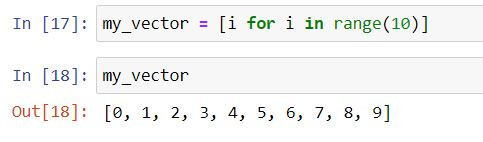Search
•Ekta Aggarwal

# List comprehensions in Python

In Python there exists a more elegant way to create new lists using already existing lists i.e. list comprehensions.

If we only want to create news lists using existing lists then list comprehensions provide easy readability with removing the necessity of writing long for loops and if else. Moreover it is quite efficient in terms of run time.

### Basic syntax of a list comprehension:

new_list = [computation FOR iterator IN exisiting_list]

### Understanding with examples:

To understand more about list comprehensions let us take an example. Firstly we are creating a list of scores.

`scores = [50,55,74,12,63]`

Task: Covert the scores in percentage by dividing them by 100 and store the result in a new list called pct.

To accomplish this task without list comprehensions we will firstly create an empty list and then would append the output using append( ) function in a for loop.

```pct = []
for i in scores:
pct.append(i/100)

pct```Using list comprehension: We need to simply write:

```pct2 = [i/100 for i in scores]
pct2```

where pct2 is our new object,

i/100 is our computation expression i.e.

i is our iterator variable

scores is our existing list.When the loop runs for the first time then i takes the value 50 and resultant computation is 50/100 i.e. 0.5, then i takes the value 55 and result gets stored as 0.55 and so on.

Here our for loop works for each element in scores list (keeps iterator value as i) and the resultant output is i/100 .

## IF ELSE in List comprehensions

### Case 1: Saving the output when a particular condition gets satisfied!

Syntax:

new_list = [output FOR iterator IN existing_list IF condition]

Task: Store the output in pct2 only if the score is more than 33.

To achieve this in our previous list comprehension we add the condition after for loop: IF i > 33

```pct2 = [i/100 for i in scores if i >33]
pct2```### Case 2: Creating a new list on the basis of IF ELSE conditions!

Syntax:

new_list = [output (when IF condition is true) IF condition ELSE output(when IF condition is false) FOR iterator IN existing_list]

Task: Store the output in pct2 as follows: if the score is more than 33 then score/100, otherwise Fail.

For this we have defined our condition in IF statement as i > 33.

Note we have specified the output of IF condition before writing IF keyword i.e. i/100.

Output for ELSE condition is written after ELSE keyword.

and lastly we write the for loop in syntax.

```pct2 = [i/100 IF i > 33 ELSE "Fail" FOR i IN scores]
pct2```Explanation:

Here for loop works first (although it is written in the end). For each i it checks if i >33 or not. For i = 50 IF else condition is evaluated, since i > 33 then 50/100 is the output, then eventually when it reaches i = 12 IF conditions get negated and then it shows the ELSE output i.e. Fail.

### List comprehension using range( ) function

range( ) is Python's inbuilt function to which creates a list.

In the following example range(10) results in a list of numbers from 0 to 9 (10 is excluded).

Using list comprehensions we are creating a new list my_vector using range( ) function.

```my_vector = [i for i in range(10)]
my_vector```## Multiple for loops in list comprehension

A list can have elements which are either a tuple or a list or elements.

Task: Create a list where elements are a tuple of two elements:

first element: tuple ranging from 0 to 1 and

second element: tuple ranging from 5 to 6.

Without list comprehensions we would have created two for loops for two different ranges and would have appended the output as follows:

```results = []

for temp1 in range(0,2):
for temp2 in range(5,7):
results.append((temp1,temp2))

results

```Using list comprehensions: We can simply avoid writing so many lines of codes and can achieve the same output in less time:

```results2 = [(temp1,temp2) for temp1 in range(0,2) for temp2 in range(5,7)]
results2```Explanation:

Here firstly temp1 takes the value 0 and then temp2 takes the value 5, result gets appended to results2.

Then temp1 still has the value 0 while temp2 take the value 6 and the result gets appended.

Similarly, temp1 takes the value 1 and then temp2 takes the values 5 and 6 and we get out final list as output!

Tags:

See All